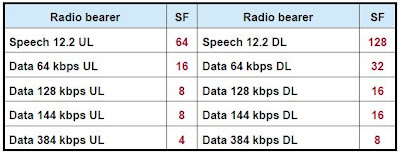TELETOPIX.ORG

Telecom Techniques Guide

WCDMA

# WCDMA Channelization Code

OVSF Code (Orthogonal Variable Spreading Factor) is used as channelization code• Orthogonal codes are easily generated by starting with a seed of 1, repeating the 1 horizontally and vertically, and then complementing the -1 diagonally. This process is to be continued with the newly generated block until the desired codes with the proper length are generated. Sequences created in this way are referred as “Walsh” code.
• Channelization uses OVSF code, for keeping the orthogonality of different subscriber physical channels. OVSF can be defined as the code tree illustrated in the diagram.
• Channelization code is defined as Cch SF, k,, where, SF is the spreading factor of the code, and k is the sequence of code, 0?k?SF-1. Each level definition length of code tree is SF channelization code, and the left most value of each spreading code character is corresponding to the chip which is transmitted earliest.

SF = chip rate / symbol rate
High data rates ? low SF code
Low data rates ? high SF code• The channelization codes are Orthogonal Variable Spreading Factor (OVSF) codes. They are used to preserve orthogonality between different physical channels. They also increase the clock rate to 3.84 Mcps. The OVSF codes are defined using a code tree.
• In the code tree, the channelization codes are individually described by Cch,SF,k, where SF is the Spreading Factor of the code and k the code number, 0 ? k ? SF-1.  A channelization sequence modulates one user’s bit. Because the chip rate is constant, the different lengths of codes enable to have different user data rates. Low SFs are reserved for high rate services while high SFs are for low rate services.
• The length of an OVSF code is an even number of chips and the number of codes (for one SF) is equal to the number of chips and to the SF value.
• The generated codes within the same layer constitute a set of orthogonal codes.
Furthermore, any two codes of different layers are orthogonal except when one of the two codes is a mother code of the other. For example C4,3 is not orthogonal with C1,0 and C2,1, but is orthogonal with C2,0.
SF in uplink is from 4 to 256.
SF in downlink is from 4 to 512.